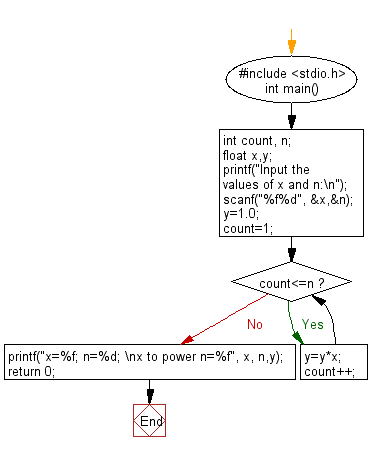﻿ C Program: Evaluate y=xn when n is non-negative

# C Exercises: Evaluate the equation y=xn when n is a non-negative integer

## C Basic Declarations and Expressions: Exercise-67 with Solution

Write a C program to evaluate the equation y=xn when n is a non-negative integer.

Sample Solution:

C Code:

``````#include <stdio.h>
int main(){
int count, n;
float x,y;

// Input the values of x and n
printf("Input the values of x and n:\n");
scanf("%f%d", &x,&n);

y=1.0;
count=1;

// Calculate x to the power n
while(count<=n)
{
y=y*x;
count++;
}

// Print the result
printf("x=%f; n=%d; \nx to power n=%f", x, n,y);

return 0;
}
``````

Sample Output:

```Input the values of x and n: 256
x=256.000000; n=0;
x to power n=1.000000
```

Flowchart:C programming Code Editor:

What is the difficulty level of this exercise?

Test your Programming skills with w3resource's quiz.

﻿